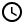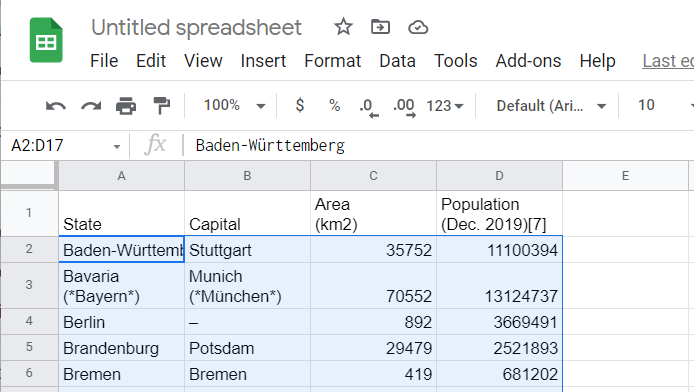# How to Sort a Table Alphabetically in Google Sheets [5 Methods!]

Sometimes, your Google Sheets data is not organized, but we are here to help. Click to discover 5 simple methods to organize your Google Sheets columns.1. How to sort a single column by right-clicking
2. How to sort a single column using a trick
3. How to sort the entire table through a selected column
4. How to use SORT function in sorting
5. How to sort using QUERY function6 Minutes

## How to sort a single column by right-clicking

If you have access to the list and you don’t mind it being modified, there is a simple method of doing so.

Step 1: Select the whole column:

Step 2: Right-click on the column header. Select Sort sheet A->Z if you want to alphabetize it; select Sort sheet Z->A if you want to reverse alphabetize the list.

The result is:

## How to sort a single column using a trick

A quick trick is to hover your cursor on the header of the column that you want to sort, then click on the appearing arrow:

The same options when you right click will appear, with an added bonus of not having to select the column first.

## How to sort the entire table through a selected column

Often, we need to sort an entire table by sorting a specific column in it. For our example below, we want to sort the table alphabetically by the data in the second column, the capitals of the states. To do so, here are the steps:

Step 1: Select the entire table:Selected range with multiple columns. We want to sort it by its second column.

Step 2: Right-click the range. Select Sort range.

Step 3: A box will appear where you can set the options for sorting.

We have options for sorting the range:

Data has header row: this is when your range includes the header row of your table. Check it when your range does; in our example, the header row is not included in our selected data.

Sort by: a drop-down box containing the columns in the selected range will appear. In our example, we want to sort the table by the alphabetical order of its capitals, which is in Column B. Therefore we select Column B.

A->Z and Z->A specifies the sort order whether in ascending or descending order, respectively.

Step 4: Click Sort.

The entire table is now sorted.

## How to use SORT function in sorting

Google Sheets has its own function for sorting: the SORT function. It has the following syntax:

=SORT(range, sort_column, is_ascending)

Where

range = the range of the table you want to sort

sort_column = the column number that you want to use for sorting. The table will be sorted based on this column. The first column is numbered 1.

is_ascending = a TRUE value will sort the list alphabetically or in ascending order. A FALSE value will sort the list in descending order. This has no default value.

This is a handy function if you want to keep the original data but want the data in the new sheet modified. For our example, the range is A2:D17, and we want to sort this in the 2nd column:

The function will now be:

=sort(A2:D17,2,TRUE)

The result is:

As you can see, the SORT function sorted first the dashes.

## How to sort using QUERY function

Finally, we can use the QUERY function to sort the list alphabetically. The syntax is:

=QUERY(range, “order by sort_column”)

Where:

range = the range of the table you want to sort

sort_column = the column number that you want to use for sorting. The table will be sorted based on this column. The first column is numbered 1.

For our example, the function will be:

=query(A2:D17,"order by B")

The result is:

As you can see, the QUERY function sorted first the letters before the dashes, unlike the case of the SORT function.

Oops! Something went wrong while submitting the form.

July 19, 2022## How to sort a single column by right-clicking

If you have access to the list and you don’t mind it being modified, there is a simple method of doing so.

Step 1: Select the whole column:

Step 2: Right-click on the column header. Select Sort sheet A->Z if you want to alphabetize it; select Sort sheet Z->A if you want to reverse alphabetize the list.

The result is:

## How to sort a single column using a trick

A quick trick is to hover your cursor on the header of the column that you want to sort, then click on the appearing arrow:

The same options when you right click will appear, with an added bonus of not having to select the column first.

## How to sort the entire table through a selected column

Often, we need to sort an entire table by sorting a specific column in it. For our example below, we want to sort the table alphabetically by the data in the second column, the capitals of the states. To do so, here are the steps:

Step 1: Select the entire table:Selected range with multiple columns. We want to sort it by its second column.

Step 2: Right-click the range. Select Sort range.

Step 3: A box will appear where you can set the options for sorting.

We have options for sorting the range:

Data has header row: this is when your range includes the header row of your table. Check it when your range does; in our example, the header row is not included in our selected data.

Sort by: a drop-down box containing the columns in the selected range will appear. In our example, we want to sort the table by the alphabetical order of its capitals, which is in Column B. Therefore we select Column B.

A->Z and Z->A specifies the sort order whether in ascending or descending order, respectively.

Step 4: Click Sort.

The entire table is now sorted.

## How to use SORT function in sorting

Google Sheets has its own function for sorting: the SORT function. It has the following syntax:

=SORT(range, sort_column, is_ascending)

Where

range = the range of the table you want to sort

sort_column = the column number that you want to use for sorting. The table will be sorted based on this column. The first column is numbered 1.

is_ascending = a TRUE value will sort the list alphabetically or in ascending order. A FALSE value will sort the list in descending order. This has no default value.

This is a handy function if you want to keep the original data but want the data in the new sheet modified. For our example, the range is A2:D17, and we want to sort this in the 2nd column:

The function will now be:

=sort(A2:D17,2,TRUE)

The result is:

As you can see, the SORT function sorted first the dashes.

## How to sort using QUERY function

Finally, we can use the QUERY function to sort the list alphabetically. The syntax is:

=QUERY(range, “order by sort_column”)

Where:

range = the range of the table you want to sort

sort_column = the column number that you want to use for sorting. The table will be sorted based on this column. The first column is numbered 1.

For our example, the function will be:

=query(A2:D17,"order by B")

The result is: